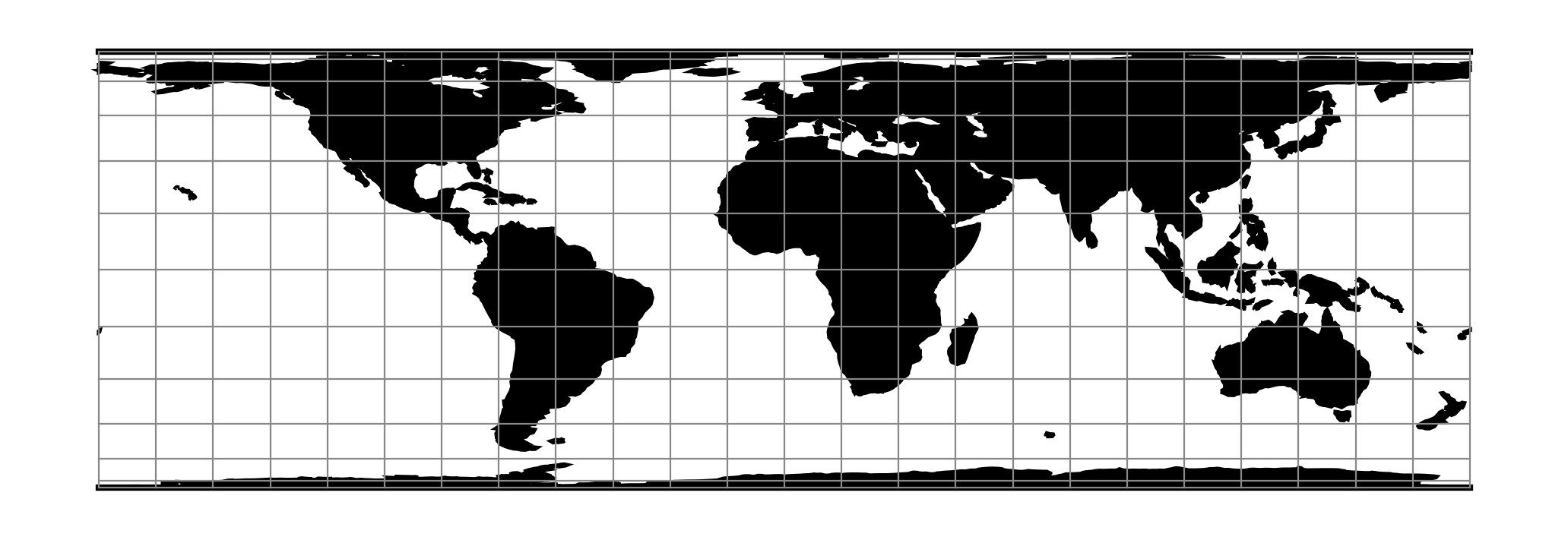# Foucaut Sinusoidal¶

 Classification Pseudocylindrical Available forms Forward and inverse, spherical projection Defined area Global Alias fouc_s Domain 2D Input type Geodetic coordinates Output type Projected coordinatesproj-string: +proj=fouc_s

The y-axis is based upon a weighted mean of the cylindrical equal-area and the sinusoidal projections. Parameter $$n=n$$ is the weighting factor where $$0 <= n <= 1$$.

\begin{align}\begin{aligned}x &= \lambda \cos \phi / (n + (1 - n) \ cos \phi)\\y &= n \phi + (1 - n) \sin \phi\end{aligned}\end{align}

For the inverse, the Newton-Raphson method can be used to determine $$\phi$$ from the equation for $$y$$ above. As $$n \rightarrow 0$$ and $$\phi \rightarrow \pi/2$$, convergence is slow but for $$n = 0$$, $$\phi = \sin^1y$$

## Parameters¶

Note

All parameters are optional for the Foucaut Sinusoidal projection.

+n=<value>

Weighting factor. Value should be in the interval 0-1.

+lon_0=<value>

Longitude of projection center.

Defaults to 0.0.

+R=<value>

Radius of the sphere given in meters. If used in conjunction with +ellps +R takes precedence.

+x_0=<value>

False easting.

Defaults to 0.0.

+y_0=<value>

False northing.

Defaults to 0.0.